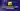Hey, psst! Do you want to grow into Web Development? I've something for you.
<->In this article, you will discover three ways to do an email validation with JavaScript (using Regular Expressions, an Email Validator library, or HTML5).

"An old myth says that you are a web development wizard once you create a contact form! 🧙😄" - Unknown

## Email validation using Regular Expressions

The most common way to validate an email with JavaScript is to use a regular expression (RegEx). Regular expressions will help you to define rules to validate a string.

If we summarize, an email is a string following this format:

1. First part of an email

• uppercase and lowercase letters: a-z and A-Z
• digits: 0-9
• hyphens: - (not the last or first character)
• dots: . (not the last or first character)

Note: Some email providers allows email adresses with these character: `! # \$ % & ‘ \* + / = ? ^ \ _ \ { | } ~ " ( ) , : ; < > @ [ \ ]`. It will depends if you want to accept these mails, but most website rejects them.

2. Second part of an email

• uppercase and lowercase letters: a-z and A-Z
• digits: 0-9
• hyphens: - (not the last or first character)

3. Third part of an email

• uppercase and lowercase letters: a-z and A-Z
• digits: 0-9
• dots: . (not the last or first character)

Here is an email regex expression:

``/^[a-zA-Z0-9][\-_\.\+\!\#\\$\%\&\'\*\/\=\?\^\`\{\|]{0,1}([a-zA-Z0-9][\-_\.\+\!\#\\$\%\&\'\*\/\=\?\^\`\{\|]{0,1})*[a-zA-Z0-9]@[a-zA-Z0-9][-\.]{0,1}([a-zA-Z][-\.]{0,1})*[a-zA-Z0-9]\.[a-zA-Z0-9]{1,}([\.\-]{0,1}[a-zA-Z]){0,}[a-zA-Z0-9]{0,}\$/i``

In JavaScript, you can use this function for your email validation.

``````function isEmailValid(email) {
const emailRegexp = new RegExp(
/^[a-zA-Z0-9][\-_\.\+\!\#\\$\%\&\'\*\/\=\?\^\`\{\|]{0,1}([a-zA-Z0-9][\-_\.\+\!\#\\$\%\&\'\*\/\=\?\^\`\{\|]{0,1})*[a-zA-Z0-9]@[a-zA-Z0-9][-\.]{0,1}([a-zA-Z][-\.]{0,1})*[a-zA-Z0-9]\.[a-zA-Z0-9]{1,}([\.\-]{0,1}[a-zA-Z]){0,}[a-zA-Z0-9]{0,}\$/i
)

return emailRegexp.test(email)
}

console.log(isEmailValid('[email protected]')) // true
console.log(isEmailValid('[email protected]')) // true
console.log(isEmailValid('[email protected]')) // true
console.log(isEmailValid('[email protected]')) // true
console.log(isEmailValid('[email protected]')) // false
console.log(isEmailValid('[email protected]')) // false
console.log(isEmailValid('@herewecode.io')) // false
console.log(isEmailValid('helloitsmeherewecode.io')) // false
console.log(isEmailValid('[email protected]')) // false
console.log(isEmailValid('[email protected]')) // false``````

Note: As you can imagine, this Regex isn't homemade. I found it on Stack Overflow; then, I updated it to match the email string format explained above.

## Email validation using an Email Validator

If you don't want to create a custom function to validate emails, you can use libraries. When we type: "email validation library javascript" on Google, the first result is the "email validator" library.

Here is an example of how to use it:

``````const validator = require('email-validator')

console.log(validator.validate('[email protected]')) // true
console.log(validator.validate('[email protected]')) // true
console.log(validator.validate('[email protected]')) // true
console.log(validator.validate('[email protected]')) // true
console.log(validator.validate('[email protected]')) // false
console.log(validator.validate('[email protected]')) // false
console.log(validator.validate('@herewecode.io')) // false
console.log(validator.validate('helloitsmeherewecode.io')) // false
console.log(validator.validate('[email protected]')) // false
console.log(validator.validate('[email protected]')) // false``````

Email validator is one library among others. You can find a lot of them with different features online.

## Email validation using HTML5 input validation

The last way to validate an email is to use an HTML5 email input.

``<input type="email" id="email" name="email" placeholder="email" />``

Here is an example of an email validation using a simple form:

``````<!DOCTYPE html>
<html>
<body>
<h1>JavaScript Validate Email</h1>

<p>Write your email and we will validate it:</p>

<form>
<input type="email" id="email" name="email" placeholder="Email" />
<input type="submit" value="Submit" />
</form>
</body>
</html>``````